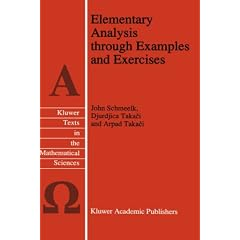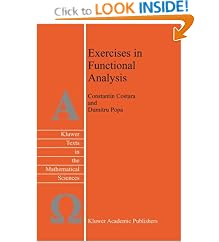# Ngô Quốc Anh

## January 31, 2009

### Problems in Real Analysis: Advanced Calculus on the Real Axis

Filed under: Các Bài Tập Nhỏ, Giải Tích Cổ Điển, Sách Hay — Ngô Quốc Anh @ 15:24

Problems in Real Analysis: Advanced Calculus on the Real Axis features a comprehensive collection of challenging problems in mathematical analysis that aim to promote creative, nonstandard techniques for solving problems. This self-contained text offers a host of new mathematical tools and strategies which develop a connection between analysis and other mathematical disciplines, such as physics and engineering. A broad view of mathematics is presented throughout; the text is excellent for the classroom or self-study. It is intended for undergraduate and graduate students in mathematics, as well as for researchers engaged in the interplay between applied analysis, mathematical physics, and numerical analysis.Key features:

• Uses competition-inspired problems as a platform for training typical inventive skills;
• Develops basic valuable techniques for solving problems in mathematical analysis on the real axis and provides solid preparation for deeper study of real analysis;
• Includes numerous examples and interesting, valuable historical accounts of ideas and methods in analysis;
• Offers a systematic path to organizing a natural transition that bridges elementary problem-solving activity to independent exploration of new results and properties.

## December 2, 2008

### Differential and Integral Equations through Practical Problems and Exercises (Texts in the Mathematical Sciences)

Filed under: Sách Hay — Ngô Quốc Anh @ 22:27This volume presents a valuable and comprehensive collection of problems and exercises, together with guidelines and worked solutions, of a wide range of the most important commonly occurring differential and integral equations.

The book is divided into two parts. Part I, which comprises eight chapters, contains the problems and exercises, and Part II, having eight corresponding chapters, presents the worked solutions. The various chapters deal, respectively, with: (1) the classical type of differential equations of first and higher order; (2) problems relating to existence and uniqueness theorems; (3) linear differential equations; (4) the method of the Laplace transform; (5) integral and integro-differential equations; (6) numerical and approximation methods; and (7) first-order partial differential equations. The final chapter (8) presents a miscellaneous selection of problems and exercises dealing with the deepest areas of differential, integral and partial differential equations.

Many of the problems discussed are original, and all have been tried and tested in a teaching environment.
This volume provides an invaluable guide to the solving of differential and integral equations and can be recommended to all researchers, students and teachers, whose work involves this fundamental aspect of mathematics.

• Hardcover: 412 pages
• Publisher: Springer; 1 edition (August 31, 1992)
• Language: English
• ISBN-10: 0792318900
• ISBN-13: 978-0792318903
• Product Dimensions: 9.2 x 6.1 x 1 inches

Tôi có bản copy của sách này…

## November 5, 2008

### Elementary Analysis through Examples and Exercises (Texts in the Mathematical Sciences)

Filed under: Sách Hay — Ngô Quốc Anh @ 14:03

This volume on mathematical analysis offers a comprehensive set of both traditional and new examples and exercises with detailed solutions. It includes many topics important in current research that are not found in other basic analysis books.It begins with a comparison of real numbers viewed as a totally ordered field or, alternatively, constructed using the Dedekind cut method. Properties surrounding real numbers are explored and many interesting relationships are proven using mathematical induction. Functions are then developed with special emphasis on topics such as asymptotics, n-levels of composition and periodicity of certain functions. Sequences and series for both the discrete and continuous case are concurrently developed showing contrast wherever possible. The order of growth for sequences diverging to infinity is incorporated with its counterpart given for functions. The usual properties of functions, together with their limit theory including the differential calculus are compared carefully with examples illustrating their fundamental properties. The graphs of these functions are then closely studied giving as much detail as possible for a wide variety of functions.

Audience: Undergraduate and graduate students in all areas of mathematical science and its applications.

• Hardcover: 332 pages
• Publisher: Springer; 1 edition (June 30, 1995)
• Language: English
• ISBN-10: 079233597X
• ISBN-13: 978-0792335979
• Product Dimensions: 9.2 x 6.1 x 0.8 inches

Tôi có bản copy của cuốn sách này..

### Complex Analysis through Examples and Exercises (Texts in the Mathematical Sciences)

Filed under: Sách Hay — Ngô Quốc Anh @ 14:00

This volume on complex analysis offers an exposition of the theory of complex analysis via a comprehensive set of examples and exercises. The book is self-contained and the exposition of new notions and methods is introduced step by step. A minimal amount of expository theory is included at the beginning of each section in the Preliminaries, with maximum effort placed on well-selected examples and exercises capturing the essence of the material.The examples contain complete solutions and serve as a model for solving similar problems given in the exercises. The readers are left to find the solution in the exercises; the answers, and occasionally, some hints, are given. Special sections contain so-called Composite Examples which consist of combinations of different types of examples explaining some problems completely and giving the reader an opportunity to check all his previously accepted knowledge.

Audience: This volume is intended for undergraduate and graduate students in mathematics, physics, technology and economics interested in complex analysis.

• Hardcover: 352 pages
• Publisher: Springer; 1 edition (July 31, 1999)
• Language: English
• ISBN-10: 0792357876
• ISBN-13: 978-0792357872
• Product Dimensions: 9.4 x 6.1 x 1 inches

Cuốn sách này có file điện tử..

### Exercises in Functional Analysis (Texts in the Mathematical Sciences)

Filed under: Sách Hay — Ngô Quốc Anh @ 13:58

This book of exercises in Functional Analysis contains almost 450 exercises (all with complete solutions), providing supplementary examples, counter-examples and applications for the basic notions usually presented in an introductory course in Functional Analysis.It contains three parts. The first one contains exercises on the general properties for sets in normed spaces, linear bounded operators on normed spaces, reflexivity, compactness in normed spaces, and on the basic principles in Functional Analysis: the Hahn-Banach theorem, the Uniform Boundedness Principle, the Open Mapping and the Closed Graph theorems.

The second one contains exercises on the general theory of Hilbert spaces, the Riesz representation theorem, orthogonality in Hilbert spaces, the projection theorem and linear bounded operators on Hilbert spaces. The third one deals with linear topological spaces, and includes a large number of exercises on the weak topologies.

• Hardcover: 451 pages
• Publisher: Springer; 1 edition (September 30, 2003)
• Language: English
• ISBN-10: 1402015607
• ISBN-13: 978-1402015601
• Product Dimensions: 9.2 x 6.3 x 1.3 inches

Tôi có bản photocopy (không phải eBook) của sách này…

## August 18, 2008

### Partial Differential Equations through Examples and Exercises (Texts in the Mathematical Sciences)

Filed under: Sách Hay — Ngô Quốc Anh @ 12:47This book examines the complicated subject of Partial Differential Equations (PDEs). It involves the reader throughout by presenting theory, examples and exercises together. Both the classical and abstract aspects of the theory are dealt with, so that, for example, classical and generalised solutions in Sobolev and distribution spaces are treated. Most of the work is devoted to second or higher order PDEs; part of the distribution theory is included, covering Dirac’s delta distribution delta function. Many practical tools are offered for solving important problems with the basic three PDEs, namely the wave equation, the Laplace equation, the heat equation and their generalisations. The majority of the problems are mathematical in character, though often physical interpretations are given.
Audience: This volume is intended for undergraduate and graduate students in mathematics, physics technology and economics interested in PDEs for modelling complex systems
• Hardcover: 416 pages
• Publisher: Springer; 1 edition (October 31, 1997)
• Language: English
• ISBN-10: 0792347242
• ISBN-13: 978-0792347248
• Product Dimensions: 9.3 x 6.3 x 1.3 inches

Tôi có bản copy của sách này…